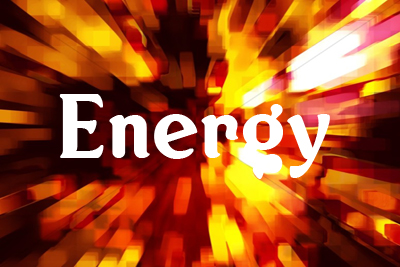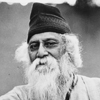FREE ASSISTANCE FOR THE INQUISITIVE PEOPLE
Tutorial Topics
Power Potential Energy - Fundamentals
Energy - Fundamentals - Work, Power and Energy
1241    Arnab De    06/09/2018### Energy

A body capable to doing work is said to possess energy. The energy possessed by a body is measured by the amount of work that the body can perform. When a body does work, its energy decreases, while if work is done on the body, its energy increases. This means that if work is done, there is always a transfer of energy. Thus energy and work are related to each other. Energy is a scalar quantity. We can define energy as follows:

Def. The energy of the body is its capacity to do work

### Unit of the Energy:

Unit of the energy in S.I system is newton-meter or joule. And the C.G.S unit of energy is erg. The bigger unit of the energy is Kilowatt hour (kWh).

W (watt) and kW(kilowatt) are the unit of power, while Wh (watt hour) and kWh(kilowatt hour) are the units of work or energy since power ✕ time = work or energy.

• Heat energy is usually measured in calorie.
• The atomic particles is very small, it is measured in electron volt (eV).

What is the difference between Energy and Power

 Energy Power Energy of the body is its capacity to do work. Power of the source is the rate at which energy is supplied by it. Energy spent does not depend on time. Power depends on the time in which energy is spent. S.I unit of energy is joule(J) S.I unit of power is watt(W)

### Mechanical Energy

The capacity of do mechanical work of a body is known as Mechanical energy of the body.

That mechanical energy are two types (i) Potential Energy and (ii) Kinetic Energy

Which will be briefly describe in the later post

Power Potential Energy - Fundamentals
Author DetailsArnab De
I have over 16 years of experience working as an IT professional, ranging from teaching at my own institute to being a computer faculty at different leading institute across Kolkata. I also work as a web developer and designer, having worked for renowned companies and brand. Through tutorialathome, I wish to share my years of knowledge with the readers.
Enter New Comment
Comment History
No Comment Found Yet.Depth of friendship does not depend on length of acquaintance.
Rabindranath Tagore
2012
25.81
Today So Far
Total View (Lakh)